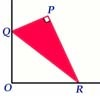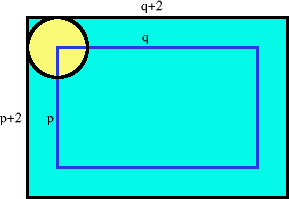#### You may also like### Set Square

A triangle PQR, right angled at P, slides on a horizontal floor with Q and R in contact with perpendicular walls. What is the locus of P?### Mach Attack

Have you got the Mach knack? Discover the mathematics behind exceeding the sound barrier.### Construct the Solar System

Make an accurate diagram of the solar system and explore the concept of a grand conjunction.

# Five Circuits, Seven Spins

##### Age 16 to 18 Challenge Level:

Alex from Stoke on Trent Sixth Form College, Manuele from the British School Manila and Dapeng Wang from Claremont Fan Court School all sent excellent solutions to this problem, well done!

This is how Alex describes how he visualised the rolling disc:

I am considering the position of the centre of the plate on the tray. At the corners of the tray, the centre of the plate stops movement in its direction and starts movement in the perpendicular direction. The plate continues to rotate anticlockwise. The distance the centre of the plate traverses is therefore identical to the distance required to rotate the plate by the same amount if it was being rolled across a single path in a single direction. If the plate was being rolled across a single path in a single direction, it would take a length of $2\pi$ for it to complete a revolution. Therefore it would take a length of $14\pi$ to complete seven revolutions, and this is equal to the distance covered by five cycles.The distance the centre traverses in 5 cycles is 5 times the perimeter of the blue line, that is $5(2p+2q)$. This is equal to $14\pi$ so $p + q = 7\pi/5$. (Where $a = p+2$ and $b= q+2$ are the dimensions of the tray).

This puts the restriction on $a$, namely $2 < a < 2 + 7\pi/5$. For every value of $a$ in this range, there exists a corresponding $b$ value for which seven revolutions are completed exactly in five cycles. This $b$ value in terms of the first length $a$ is: $b = 4 + 7\pi/5 - a.$ Therefore there is no limit to the number of solutions in this range.

The area of the tray is $ab$. Substituting the expression for $b$ in terms of $a$ in gives:

Area $A= a(4 + 7pi/5 - a)$. The derivative of this: $dA/da = 4 + 7pi/5 - 2a$ . When $dA/da = 0$ the value of $a$ is $a = 2 + 7\pi/10$, and the second derivative $d^2A/da^2 = -2$ (i.e. negative) so $a = 2 + 7\pi/10$ gives is a maximum point. The maximum area of the tray occurs when $a$ and $b$ are both $2 + 7\pi/10$, ie a square tray, and the maximum area is area $\approx 17.63$ square units.

Editorial comment: It was a nice idea to find the area of the tray. The maximum area for a rectangle with a given perimeter always occurs when the rectangle is a square. You might like to try to generalise this problem to cases where the unit disc rolls periodically inside trays of other shapes with a given number of revolutions for a given number of circuits.